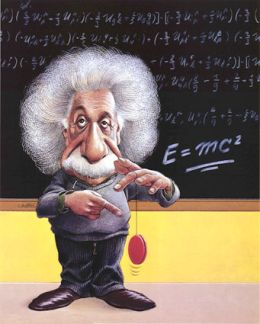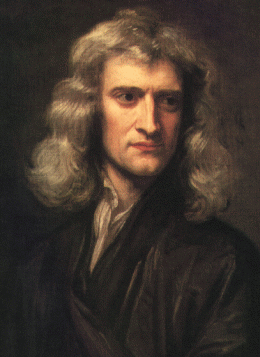IF IT'S EDUCATION, IT'S ITS
Pathways To Learning... Since 2005
Hong Kong Registered School 566985

# Physics resources for IGCSE or GCSE students.

[Science home]

Revision sheets for IGCSE or GCSE physics. This series revision sheets are based on the IGCSE & GCSE syllabuses. Lots of formulas, definitions and key points to learn off. There are also accompanying test sheets to check your memorisation.

The idea is read the information and than print the quiz sheet and try the questions.Revision Exercise one:

Forces and Motion

unit of Distance: m, "metre".

unit of time: s, "second"

Speed = Distance ÷ Time

Velocity is speed in a certain direction.

Velocity = Distance ÷ Time

unit of Velocity: m/s, "metres per second"

Acceleration: a change in velocity.

Acceleration = Change in Velocity ÷ Time.

a = (v-u) ÷ t

a = acceleration

v = final velocity

u = initial velocity

t = time

unit of acceleration:  , "metres per second squared"

Distance is the area under the velocity time graph.Newtons laws

1) Velocity remains constant unless acted on by a resultant force

2) Force = mass x acceleration

F = m x a

3) If A applies a force to B, B applies an equal but opposite force on A

Unit of force: N, "Newton"  N=kg

Mass (m) is an amount of substance. Unit kg "kilograms".

Gravity is a force of attraction between masses.

The acceleration due to gravity on earth (g) is aprox. 10 m/s2

Weight (W) is the force of gravity pulling on a mass.

W = mg

Friction is a force that opposes motion due to passing objects rubbing off each other.

Density = mass ÷ volume

= m ÷ v

Quiz for revision exercise one: Forces and motion quiz

Genius is one percent inspiration and ninety-nine percent perspiration.